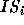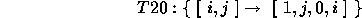Next: 5 Presburger Arithmetic with Up: The Omega Calculator Previous: 3.4 Relational and set

# 4 Code Generation

The Omega Calculator incorporates an algorithm for generating code for multiple, overlapping iteration spaces [KPR95]. Each iteration space has an associated statement or block of statements. The syntax is codegen [ effort][ given known] Each iteration spacecan be specified either as a set representing the iteration space, or as an original iteration space and a transformation, T: IS, where IS is the original iteration space and T is a relation defining an affine, 1-1 mapping to a new iteration space. That is, given a point in the original iteration space, the mapping specifies the point in the new iteration space at which to execute that iteration.

The effort value specifies the amount of effort to be used to eliiminate sources of overhead in the generated code. Sources of overhead include if statements an min and max functions in loop bounds. If not specified, the effort level is 0. The different effort levels are:

-2 Minimal possible effort. Loop bounds may not be finite.
-1 Forces finite bounds
0 Forces finite bounds and tries to remove if's within most deeply nested loops (at a possible cost of code duplication).
1 removes if's within most deeply nested loops and loops one short of being most deeply nested.
2and loops two short of being most deeply nested.
xand loops x short of being most deeply nested.

The known information, if specified, is used to simplify the generated code. The generated code will not be correct if known is not true. Currently, the known relation needs to be a set with the same arity and the transformed iteration space.

A discussion of program transformations using this framework is given in [KP93,KP94b,KP94a].

The following is an example of code generation, given three original iteration spaces and mappings. The transformed code requires the traditional transformations loop distribution and imperfectly nested triangular loop interchange. Below, the program information has been extracted and presented to the Omega Calculator in relation form.

Original code

```      do 30 i=2,n
10      sum(i) = 0.
do 20 j=1,i-1
20        sum(i) = sum(i) + a(j,i)*b(j)
30      b(i) = b(i) - sum(i)
```

Schedule (for parallelism)Omega Calculator output:

```# Omega Calculator [v1.00, Mar 96]:
# #
# # Example of code generation from Omega Calculator documentation
# #
#
# T10:={[i] -> [0,i,0,0]};
#
# T20:={[i,j] -> [1,j,0,i]};
#
# T30:={[i] -> [1,i-1,1,0]};
#
#
# Symbolic n;
#
# IS10 := {[i]: 2 <= i <= n};
#
# IS20 := {[i,j]: 2 <= i <= n && 1 <= j <= i-1};
#
# IS30 := IS10;
#
#
# codegen T10:IS10,T20:IS20,T30:IS30;
for(t2 = 2; t2 <= n; t2++) {
s1(t2);
}
for(t2 = 1; t2 <= n-1; t2++) {
for(t4 = t2+1; t4 <= n; t4++) {
s2(t4,t2);
}
s3(t2+1);
}
```Next: 5 Presburger Arithmetic with Up: The Omega Calculator Previous: 3.4 Relational and set

omega@cs.umd.edu

Web Accessibility